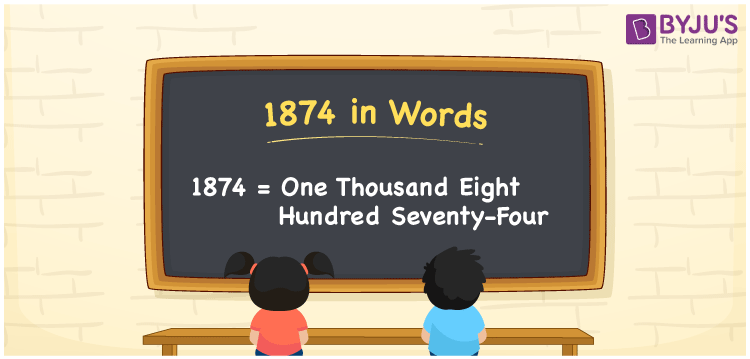# 1874 in Words

1874 in words can be written as One Thousand Eight Hundred Seventy-four. Students will be able to learn the conversion of 1874 in words which will help them understand the applications of numbers in our daily lives. If you buy groceries for Rs. 1874, then you can say that “I have bought a groceries for One Thousand Eight Hundred Seventy-four Rupees”. The  number 1874 can be written in words using the English alphabet. The numbers in words can be grasped easily by the students using the resources given at BYJU’S. 1874 in English can be read as “One Thousand Eight Hundred Seventy-four”.

 1874 in words One Thousand Eight Hundred Seventy-four One Thousand Eight Hundred Seventy-four in Numbers 1874

## 1874 in English Words## How to Write 1874 in Words?

Students will learn about the conversion of 1874 into words from place value charts. The number 1874 has four digits. For 1874, the place value chart is prepared in a table form to help students understand it effectively.

 Thousands Hundreds Tens Ones 1 8 7 4

1874 in expanded form is explained in brief here:

1 × Thousand + 8 × Hundred + 7 × Ten + 4 × One

= 1 × 1000  + 8 × 100 + 7 × 10 + 4 × 1

= 1000 + 800 + 70 + 4

= 1874

= One Thousand Eight Hundred Seventy-four

Therefore, 1874 in words is written as One Thousand Eight Hundred Seventy-four.

1874 is a natural number that precedes 1875 and succeeds 1873.

1874 in words – One Thousand Eight Hundred Seventy-four

Is 1874 an odd number? – No

Is 1874 an even number? – Yes

Is 1874 a perfect square number? – No

Is 1874 a perfect cube number? – No

Is 1874 a prime number? – No

Is 1874 a composite number? – Yes

## Frequently Asked Questions on 1874 in Words

Q1

### How do you write 1874 in words?

1874 can be written as “One Thousand Eight Hundred Seventy-four” in words.
Q2

### Is 1874 an even number?

1874 is an even number because it is divisible by 2.
Q3

### How can One Thousand Eight Hundred Seventy-four be written in numbers?

One Thousand Eight Hundred Seventy-four can be written in numbers as 1874.